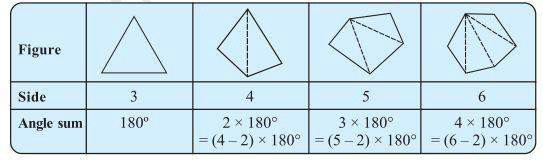# Examine the table. (Each figure is divided into triangles and the sum of the angles deduced from that.) What can you say about the angle sum of a convex polygon with number of sides? (a) 7 (b) 8 (c) 10 (d) nFrom the above given table we get to know that for a given number of sides

The sum of the angles of a convex polygon is (n – 2) × 180°.

a) 7

Ans: (7 – 2) × 180° = 5 × 180° = 900°

b) 8

Ans: (8 – 2) × 180° = 6 × 180° = 1080°

c) 10

Ans: (10 – 2) × 180° = 8 × 180° = 1440°

d) n

Ans: (n – 2) x 180°